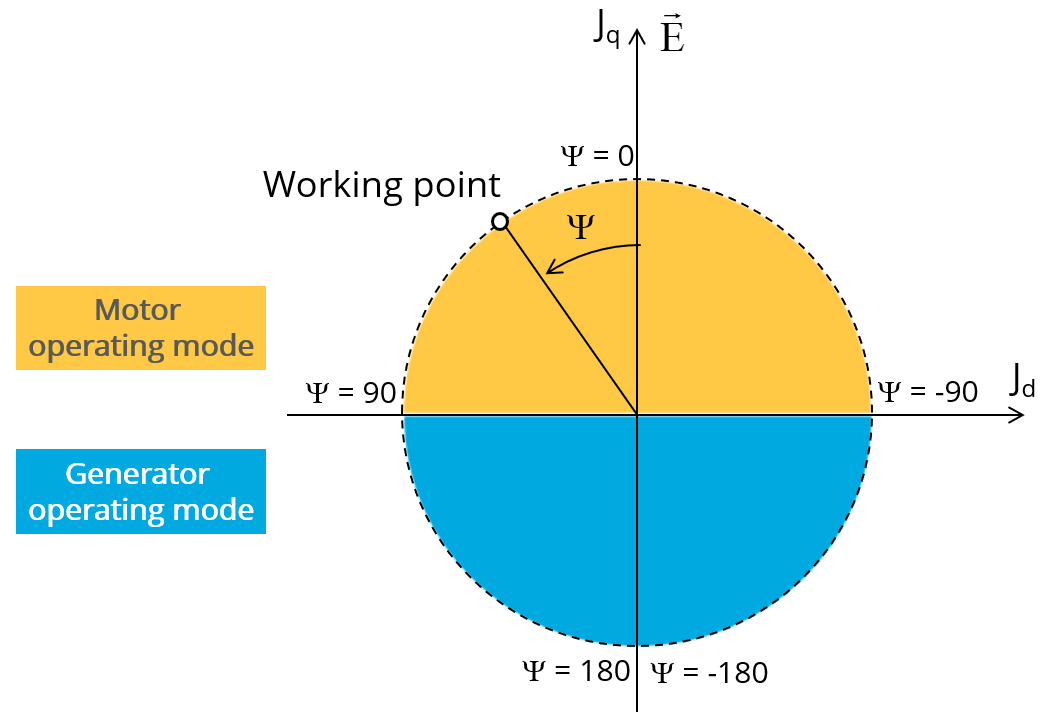# Inputs

## 1. Standard inputs

1. Line current, rms

The line current supplied to the machine: “Line current, rms” ( Line current, rms value ) must be provided.

2. Control angle

Considering the vector diagram shown below, the “ Control angle ” is the angle between the electromotive force E and the electrical current (J) (Ψ = (E, J)).

It is an electrical angle. The default value is 45 degrees. It must be set in a range of -90 to 90 degrees.

This range of values covers all the possible working point in motor convention.Definition of the control angle Ψ - Motor convention
3. Speed

The imposed “ Speed ” ( Speed ) of the machine must be set.

The list of advanced inputs dedicated to this export are listed below.

For more details please refer to the list of generic advanced inputs.

1. Number of computations per electrical period

The default value is equal to 50. The minimum allowed value is 13.

2. Number of computed electrical periods

The default value is equal to 2. The minimum allowed value is 1 and the maximum value is equal to 10.

3. Rotor initial position mode

By default, the “ Rotor initial position mode ” is set to “ Auto ”.

4. Rotor initial position
5. Mesh order

The default level is second order mesh.

6. Airgap mesh coefficient

Airgap mesh coefficient is set to 1.5 by default.